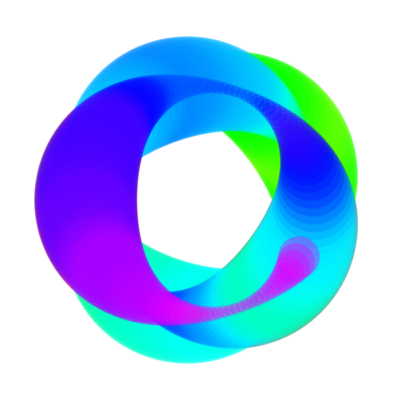# Recurrent Expressions – Outtakes #3 [loops]

Draw, move, repeat.

The [loops] sketch is about exploring the emergent shapes and patterns of a big-ish number of ellipses, adjusting size and color parameters and setting sine and cosine functions for placement on canvas.

For some reason is very satisfying to put a bunch of circles in a geometric path and let them change color and size.

It’s nice to work with color for a change! 🌈

```  ```

int loop = 500;
float r = 70;
float offsetX, offsetY, a, a2, n;

void setup() {
size(700, 700);
colorMode(HSB);
}

void draw() {
background(255);
noStroke();

for (int i = 0; i < loop; i++) {
n += 0.03;
a += 2;
a2 += 3;
//r = map(sin(radians(a)), -1, 1, 120, 20);
//offset = map(noise(n), 0, 1, -50, 50);

r = map(sin(radians(a2)), -1, 1, 50, 150);
offsetX = sin(radians(a + (i * 0.02))) * 200;
offsetY = cos(radians(a + (i * 0.09))) * 200;

fill(map(i, 0, loop, 180, 140) + map(noise(n),
0, 1, -50, 50), 255, 255, 50);
ellipse((height / 2) + offsetX, (width / 2)
+ offsetY, r, r);
}

//save("loop_" + int(random(999)) + ".png");
noLoop();
}  ```
```

The reason behind organizing and ultimately self-publishing the collection of graphic experiments Expresiones Recurrentes was to curate a little more than a year of iterations on visual sketches in Processing.

Of course a lot of material was left out of the final release, so I thought that sharing some of the results bundled up in different posts could be a way of breathing life into some of the pictures that were produced.